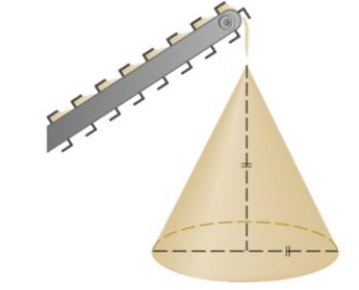Chapter 3.9, Problem 29E### Single Variable Calculus: Early Tr...

8th Edition
James Stewart
ISBN: 9781305270343

#### Solutions

Chapter
Section### Single Variable Calculus: Early Tr...

8th Edition
James Stewart
ISBN: 9781305270343
Textbook Problem

# Gravel is being dumped from a conveyor belt at a rate of 30ft 3 min, and its coarseness is such that it forms a pile in the shape of a cone whose base diameter and height are always equal. How fast is the height of the pile increasing when the pile is 10ft high?To determine

To find: The rate of change of the height of the cone shape pile whose base diameter and

height are always equal.

Explanation

Given:

Consider the gravel is being dumped from a conveyor belt at a rate as dVdt=30ft3/min.

The diameter of the base and height of the pile are always same.

Formula used:

(1) Chain rule: dydx=dydududx

(2) Volume of the cone of base radius r and height h:V=13πr2h.

Calculation:

Let V be the volume of the cone shape pile and h be the height.

Since height and diameter of the cone shape pile are always same. Thus, radius of the cone shape pile is h2 .as shown in the figure-1 given below.

Since V and h changes with the time t, V and h are the function of the time t.

Obtain dhdt when h=10ft.

Substitute r=h2 in V,

V=13π(h2)2h=112πh3

Differentiate V with respect to the time t.

ddt[V]=ddt[112πh3]dVdt=112π(3h2)dhdt

### Still sussing out bartleby?

Check out a sample textbook solution.

See a sample solution

#### The Solution to Your Study Problems

Bartleby provides explanations to thousands of textbook problems written by our experts, many with advanced degrees!

Get Started

#### 23/2

Applied Calculus for the Managerial, Life, and Social Sciences: A Brief Approach

#### The area of the region bounded by , , and r = sec θ is:

Study Guide for Stewart's Multivariable Calculus, 8th

#### limh0ah1h=1 for a = a) 0 b) 1 c) e d) The limit is never 1.

Study Guide for Stewart's Single Variable Calculus: Early Transcendentals, 8th

#### Evaluate each expression. (54)

College Algebra (MindTap Course List)